## Friction

The property by virtue of which the relative motion between two surfaces in contact is opposed is known as friction.

### Frictional Forces –

Tangential forces developed between the two surfaces in contact, so as to oppose their relative motion are known as frictional forces or commonly friction.

### Types of Frictional Forces –

Frictional forces are of three types:-

1. Static frictional force
2. Kinetic frictional force
3. Rolling frictional force

Let us return to the example of a body of mass m at rest on a horizontal table. The force of gravity (mg) is cancelled by the normal reaction force (N) of the table. Now suppose a force F is applied horizontally to the body. We know from experience that a small applied force may not be enough to move the body. But if the applied force F were the only external force on the body, it must move with acceleration F/m, however small. Clearly, the body remains at rest because some other force comes into play in the horizontal direction and opposes the applied force F, resulting in zero net force on the body. This force fs parallel to the surface of the body in contact with the table is known as frictional force, or simply friction.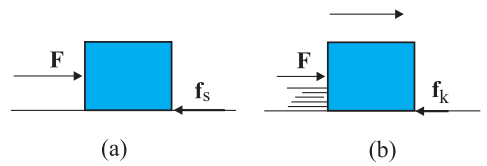In this fig. , Static and sliding friction: (a) Impending motion of the body is opposed by static friction. When external force exceeds the maximum limit of static friction, the body begins to move.

(b) Once the body is in motion, it is subject to sliding or kinetic friction which opposes relative motion between the two surfaces in contact. Kinetic friction is usually less than the maximum value of static friction fk is kinetic friction.

### Static Frictional Force-

Frictional force acting between the two surfaces in contact which are relatively at rest, so as to oppose their relative motion, when they tend to move relatively under the effect of any external force is known as static frictional force. Static frictional force is a self adjusting force and its value lies between its minimum value up to its maximum value.

### Kinetic Frictional Force-

Frictional force acting between the two surfaces in contact which are moving relatively, so as to oppose their relative motion, is known as kinetic frictional force. It’s magnitude is almost constant and is equal to µkN where µk is the coefficient of kinetic friction for the given pair of surface and N is the normal reaction acting between the two surfaces in contact. It is always less than maximum value of static frictional force.

### Minimum value of static frictional force –

Minimum value of static frictional force is zero in the condition when the bodies are relatively at rest and no external force is acting to move them relatively.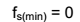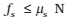?s = constant of proportionality depending only on the nature of the surfaces in contact. The constant ?s is called the coefficient of static friction.

### Maximum value of static frictional force –

Maximum value of static frictional force is µsN in the condition when the bodies are just about to move relatively under the effect of external applied force.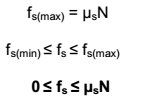### Angle of Friction:

The angle between the resultant contact force (of normal reaction and friction) and the normal reaction is known as the angle of friction.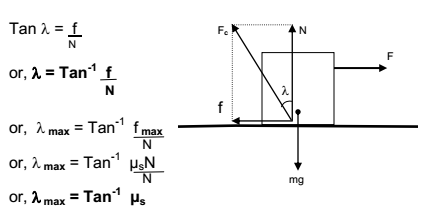### Angle of Repose:

The angle of the inclined plane at which a body placed on it just begins to slide is known as angle of repose.

Perpendicular to the plane N = mgCosθ (since body is at rest)

Parallel to the plane when body is at rest mgSinθ = fs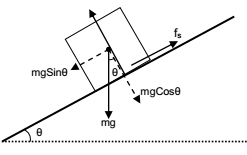When body is just about to slide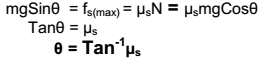Note: Angle of repose is equal to the maximum value of angle of friction

### Rolling Frictional Force:

Frictional force which opposes the rolling of bodies (like cylinder, sphere, ring etc.) over any surface is called rolling frictional force. Rolling frictional force acting between any rolling body and the surface is almost constant and is given by µr N. Where µr is coefficient of rolling friction and N is the normal reaction between the rolling body and the surface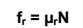Note – Rolling frictional force is much smaller than maximum value of static and kinetic frictional force.Methods to Reduce Friction

1. By polishing – Polishing makes the surface smooth by filling the space between the depressions and projections present in the surface of the bodies at microscopic level and there by reduces friction.
2. By proper selection of material – Since friction depends upon the nature of material used hence it can be largely reduced by proper selection of materials.
3. By lubricating – When oil or grease is placed between the two surfaces in contact, it prevents the surface from coming in actual contact with each other. This converts solid friction into liquid friction which is very small.

## Circular Motion

When a body moves such that it always remains at a fixed distance from a fixed point then its motion is said to be circular motion. The fixed distance is called the radius of the circular path and the fixed point is called the center of the circular path.

### Uniform Circular Motion –

Circular motion performed with a constant speed is known as uniform circular motion.

Example of Circular Motion:

1. Motion of a car on a banked road: In case of horizontal road necessary centripetal force mv2/r is provided by static frictional force. When heavy vehicles move with high speed on a sharp turn (small radius) then all the factors contribute to huge centripetal force which if provided by the static frictional force may result in the fatal accident.
To prevent this roads are banked by lifting their outer edge. Due to this, normal reaction of road on the vehicle gets tilted inwards such that it’s vertical component balances the weight of the body and the horizontal component provides the necessary centripetal force.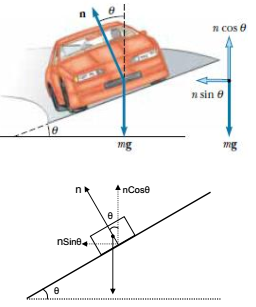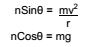Therefore,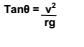### Centripetal Force

The acceleration of a body moving in a circle of radius R with uniform speed v is v2/R directed towards the centre. According to the second law, the force f providing this acceleration is: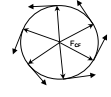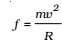where m is the mass of the body. This force directed forwards the centre is called the centripetal force.

For a stone rotated in a circle by a string, the centripetal force is provided by the tension in the string. The centripetal force for motion of a planet around the sun is the gravitational force on the planet due to the sun. For a car taking a circular turn on a horizontal road, the centripetal force is the force of friction.